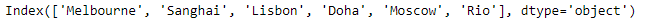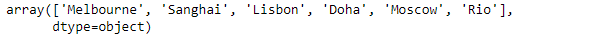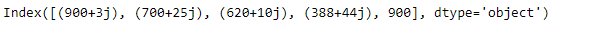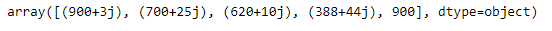GFG App
Open AppBrowser
Continue

# Python | Pandas Index.values

Pandas Index is an immutable ndarray implementing an ordered, sliceable set. It is the basic object which stores the axis labels for all pandas objects.

Pandas` Index.values` attribute return an array representing the data in the given Index object.

Syntax: Index.values

Parameter : None

Returns : an array

Example #1: Use `Index.values` attribute to return an array representing the data in the given Index object.

 `# importing pandas as pd ` `import` `pandas as pd ` ` `  `# Creating the index ` `idx ``=` `pd.Index([``'Melbourne'``, ``'Sanghai'``, ``'Lisbon'``, ``'Doha'``, ``'Moscow'``, ``'Rio'``]) ` ` `  `# Print the index ` `print``(idx) `

Output :Now we will use `Index.values` attribute to return an array representing the data in the given Index object.

 `# return an array ` `result ``=` `idx.values ` ` `  `# Print the result ` `print``(result) `

Output :As we can see in the output, the `Index.values` attribute has successfully returned an array representing the data of the given Index object.

Example #2 : Use `Index.values` attribute to return an array representing the data in the given Index object.

 `# importing pandas as pd ` `import` `pandas as pd ` ` `  `# Creating the index ` `idx ``=` `pd.Index([``900` `+` `3j``, ``700` `+` `25j``, ``620` `+` `10j``, ``388` `+` `44j``, ``900``]) ` ` `  `# Print the index ` `print``(idx) `

Output :Now we will use `Index.values` attribute to return an array representing the data in the given Index object.

 `# return an array ` `result ``=` `idx.values ` ` `  `# Print the result ` `print``(result) `

Output :As we can see in the output, the `Index.values` attribute has successfully returned an array representing the data of the given Index object.

My Personal Notes arrow_drop_up